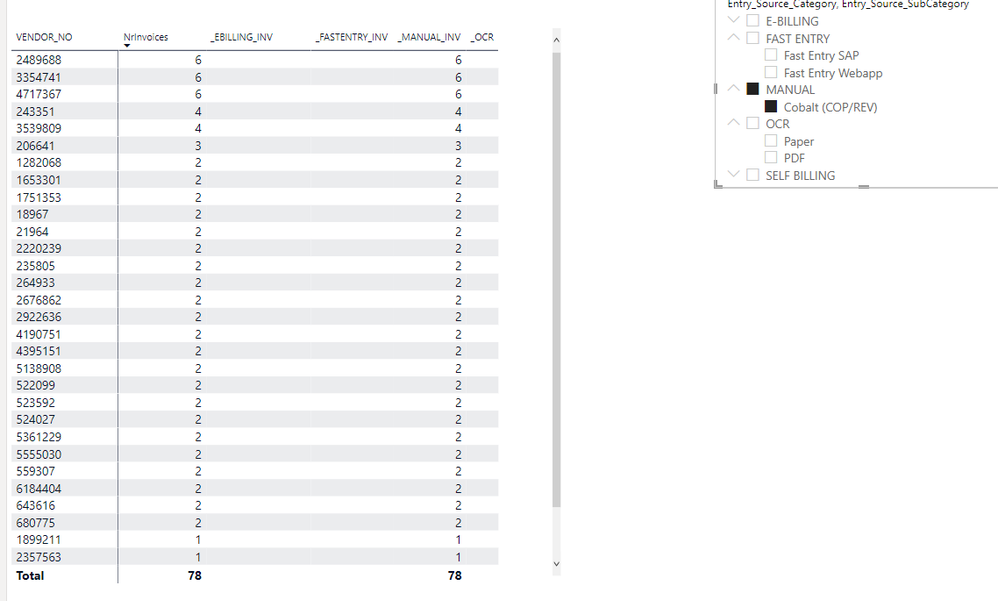cancel
Showing results for
Did you mean:Helper II

## How to fix this Slicer/Measure issue? Sample dataset provided

Hello community

I am having the bellow issue in a current report.

I have a table with XWY Dimensions and as columns I am using some measures to calculate the amount of invocies received via the different "entry source channels".

On the right side I have a slicer that I want to use it to filter the rawdata, therefore what I do not understand is why even though I filter for example "Manual" invoices I still see the values of ther other categories.A shorter version of the example can be found in my PBI file.

Thanks a lot and looking forward for your help-.

1 ACCEPTED SOLUTIONCommunity Support

Please refer to the pbix file to see if it helps you.

Modify the  measures.

``````_EBILLING_INV = VAR _1= CALCULATE(sum(Table1[NrInvoices]), Table1[Entry_Source_Category] = "E-BILLING")
RETURN
IF(HASONEVALUE(Table1[Entry_Source_Category])&&MAX(Table1[Entry_Source_Category])= "E-BILLING",_1,BLANK())``````
``````_FASTENTRY_INV = VAR _1= CALCULATE(sum(Table1[NrInvoices]),Table1[Entry_Source_Category] = "FAST ENTRY")
RETURN
IF(HASONEVALUE(Table1[Entry_Source_Category])&&MAX(Table1[Entry_Source_Category])="FAST ENTRY",_1,BLANK())``````
``````_MANUAL_INV = var _a= CALCULATE(sum(Table1[NrInvoices]),Table1[Entry_Source_Category] = "MANUAL")
return
IF(HASONEVALUE(Table1[Entry_Source_SubCategory])&&MAX(Table1[Entry_Source_Category])="MANUAL",_a,BLANK())``````
``````_OCR = VAR _1= CALCULATE(sum(Table1[NrInvoices]),Table1[Entry_Source_Category] = "OCR")
RETURN
IF(HASONEVALUE(Table1[Entry_Source_Category])&&MAX(Table1[Entry_Source_Category])="OCR",_1,BLANK())``````If I have misunderstood your meaning, provide more details with your desired output.

Best Regards

Community Support Team _ Polly

If this post helps, then please consider Accept it as the solution to help the other members find it more quickly.

4 REPLIES 4Community Support

Please refer to the pbix file to see if it helps you.

Modify the  measures.

``````_EBILLING_INV = VAR _1= CALCULATE(sum(Table1[NrInvoices]), Table1[Entry_Source_Category] = "E-BILLING")
RETURN
IF(HASONEVALUE(Table1[Entry_Source_Category])&&MAX(Table1[Entry_Source_Category])= "E-BILLING",_1,BLANK())``````
``````_FASTENTRY_INV = VAR _1= CALCULATE(sum(Table1[NrInvoices]),Table1[Entry_Source_Category] = "FAST ENTRY")
RETURN
IF(HASONEVALUE(Table1[Entry_Source_Category])&&MAX(Table1[Entry_Source_Category])="FAST ENTRY",_1,BLANK())``````
``````_MANUAL_INV = var _a= CALCULATE(sum(Table1[NrInvoices]),Table1[Entry_Source_Category] = "MANUAL")
return
IF(HASONEVALUE(Table1[Entry_Source_SubCategory])&&MAX(Table1[Entry_Source_Category])="MANUAL",_a,BLANK())``````
``````_OCR = VAR _1= CALCULATE(sum(Table1[NrInvoices]),Table1[Entry_Source_Category] = "OCR")
RETURN
IF(HASONEVALUE(Table1[Entry_Source_Category])&&MAX(Table1[Entry_Source_Category])="OCR",_1,BLANK())``````If I have misunderstood your meaning, provide more details with your desired output.

Best Regards

Community Support Team _ Polly

If this post helps, then please consider Accept it as the solution to help the other members find it more quickly.Super User

The filter for manual is being applied to the whole table, so you are only seeing vendors who have at least 1 Manul entry. Within your measures you are explicitly filtering for just the appropriate entry type, so you are showing e.g. the number of ebilling invoices for those vendors who have at least 1 Manual entry.

The numbers your measures are showing are correct, you just need to understand the context.Helper II

If my whole dataset is being filtered by the slicer why would it show the figures from data that is not selected?

How can I make it work on the way I want?

By the way the raw data is on Invoice levelSuper User

You would need to check within each measure whether there is a single value selected for the invoice type, and if it matches the relevant category, e.g.

``E-Billing # = IF( SELECTEDVALUE(Table1[Entry_Source_Category]) = "E-BILLING", CALCULATE(SUM(Table1[NrInvoices])))``